Courses

# Introduction to Turbulent Flow - 2 Civil Engineering (CE) Notes | EduRev

## Civil Engineering (CE) : Introduction to Turbulent Flow - 2 Civil Engineering (CE) Notes | EduRev

The document Introduction to Turbulent Flow - 2 Civil Engineering (CE) Notes | EduRev is a part of the Civil Engineering (CE) Course Fluid Mechanics.
All you need of Civil Engineering (CE) at this link: Civil Engineering (CE)

Reynolds decomposition of turbulent flow :

• The Experiment: In 1883, O. Reynolds conducted experiments with pipe flow by feeding into the stream a thin thread of liquid dye. For low Reynolds numbers, the dye traced a straight line and did not disperse. With increasing velocity, the dye thread got mixed in all directions and the flowing fluid appeared to be uniformly colored in the downstream flow.

The Inference: It was conjectured that on the main motion in the direction of the pipe axis, there existed a superimposed motion all along the main motion at right angles to it. The superimposed motion causes exchange of momentum in transverse direction and the velocity distribution over the cross-section is more uniform than in laminar flow. This description of turbulent flow which consists of superimposed streaming and fluctuating (eddying) motion is well known as Reynolds decomposition of turbulent flow.

• Here, we shall discuss different descriptions of mean motion. Generally, for Eulerian velocity u , the following two methods of averaging could be obtained.

(i) Time average for a stationary turbulence: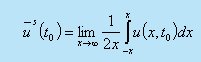(ii) Space average for a homogeneous turbulence: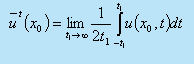For a stationary and homogeneous turbulence, it is assumed that the two averages lead to the same result: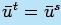and the assumption is known as the ergodic hypothesis.

• In our analysis, average of any quantity will be evaluated as a time average . Take a finite time interval t1. This interval must be larger than the time scale of turbulence. Needless to say that it must be small compared with the period t2 of any slow variation (such as periodicity of the mean flow) in the flow field that we do not consider to be chaotic or turbulent .

Thus, for a parallel flow, it can be written that the axial velocity component is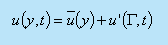(32.1)

As such, the time mean component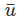(y)determines whether the turbulent motion is steady or not. The symbol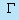signifies any of the space variables.

• While the motion described by Fig.32.6(a) is for a turbulent flow with steady mean velocity the Fig.32.6(b) shows an example of turbulent flow with unsteady mean velocity. The time period of the high frequency fluctuating component is t1 whereas the time period for the unsteady mean motion is t2 and for obvious reason t2>>t1. Even if the bulk motion is parallel, the fluctuation ' being random varies in all directions.
• The continuity equation, gives us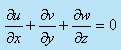Invoking Eq.(32.1) in the above expression, we get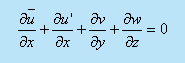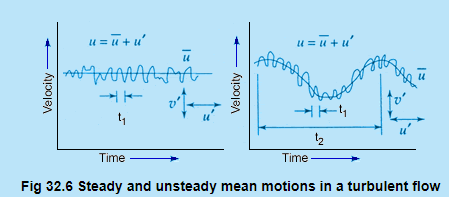Since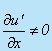, Eq.(32.2) depicts that and components of velocity exist even for the parallel flow if the flow is turbulent. We have-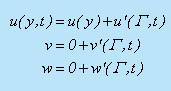(32.3)

Contd. from Previous slide

• However, the fluctuating components do not bring about the bulk displacement of a fluid element. The instantaneous displacement is U'dt , and that is not responsible for the bulk motion. We can conclude from the above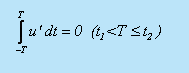Due to the interaction of fluctuating components, macroscopic momentum transport takes place. Therefore, interaction effect between two fluctuating components over a long period is non-zero and this can be expressed as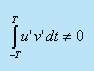Taking time average of these two integrals and write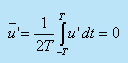(32.4a)

and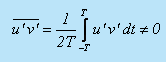(32.4b)

• Now, we can make a general statement with any two fluctuating parameters, say, with ' and g' as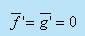(32.5a)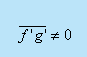(32.5b)

The time averages of the spatial gradients of the fluctuating components also follow the same laws, and they can be written as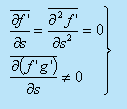(32.6)

• The intensity of turbulence or degree of turbulence in a flow is described by the relative magnitude of the root mean square value of the fluctuating components with respect to the time averaged main velocity. The mathematical expression is given by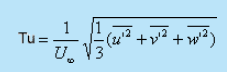(32.7a)

The degree of turbulence in a wind tunnel can be brought down by introducing screens of fine mesh at the bell mouth entry. In general, at a certain distance from the screens, the turbulence in a wind tunnel becomes isotropic, i.e. the mean oscillation in the three components are equal,In this case, it is sufficient to consider the oscillation u' in the direction of flow and to put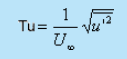(32.7b)

This simpler definition of turbulence intensity is often used in practice even in cases when turbulence is not isotropic.

Following Reynolds decomposition, it is suggested to separate the motion into a mean motion and a fluctuating or eddying motion. Denoting the time average of the u component of velocity byand fluctuating component as, we can write down the followingBy definition, the time averages of all quantities describing fluctuations are equal to zero.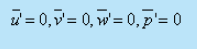(32.8)

The fluctuations u', v' , and w' influence the mean motion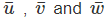in such a way that the mean motion exhibits an apparent increase in the resistance to deformation. In other words, the effect of fluctuations is an apparent increase in viscosity or macroscopic momentum diffusivity .

• Rules of mean time - averages

If and g are two dependent variables and if s denotes anyone of the independent variables x, y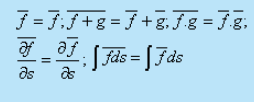Offer running on EduRev: Apply code STAYHOME200 to get INR 200 off on our premium plan EduRev Infinity!

## Fluid Mechanics

47 videos|82 docs|73 tests

,

,

,

,

,

,

,

,

,

,

,

,

,

,

,

,

,

,

,

,

,

;